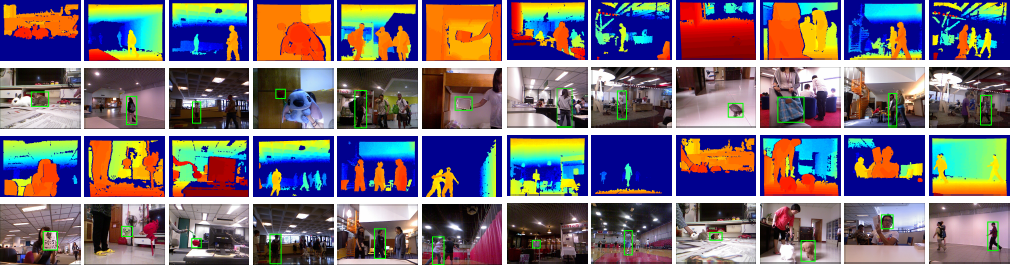Princeton Tracking Benchmark### Tracking Benchmark Datasets5 validation video with ground truth.Download here
matlab code for single test case evaluation: Download here

#### How to use the data

Each sequence folder contains following files:
1. rgb image folder : images are in 8 bit png format, named r-timestamp-frameID (e.g. r-0-1, r-1233-2);
2. depth image folder : images are in 16 bit png format, with the first 3 bit swap to the last (for visilization purpose Users need to swap them back after reading the image. Values at each pixel are the distance from Kinect to the object in mm.
3. frames.mat: contains intrinsic camera parameters: K: 3x3 intrinsic matric, fov : feild of view. Information for reading frames: video length, imageTimestamp, imageFrameID, depthTimestamp, depthFrameID. See folllowing code for details.
4. frames.json: same as frames.mat
5. init.txt: the initial bounding box for tacking: target_top_left_x,target_top_left_y,target_width,target_height
(The starting and ending frame is subject to frames.mat)
The example matlab code to read the rgb and depth frame is as follow:Download here (The 3D points are in meter now)

`````` directory = './EvaluationSet/face_occ2/';

%K is [fx 0 cx; 0 fy cy; 0 0 1];
K = frames.K;
cx = K(1,3); cy = K(2,3);
fx = K(1,1); fy = K(2,2);

numOfFrames = frames.length;
imageNames = cell(1,numOfFrames*2);
XYZcam = zeros(480,640,4,numOfFrames);

for frameId = 1:numOfFrames
imageName = fullfile(directory,sprintf('rgb/r-%d-%d.png', frames.imageTimestamp(frameId), frames.imageFrameID(frameId)));
depthName = fullfile(directory,sprintf('depth/d-%d-%d.png', frames.depthTimestamp(frameId), frames.depthFrameID(frameId)));
depth = bitor(bitshift(depth,-3), bitshift(depth,16-3));
depth = double(depth);
%show the 2D image
subplot(1,2,1); imshow(rgb);
subplot(1,2,2); imshow(depth);

%3D point for the frame
depthInpaint = depth/1000;  % convert from mm to m
[x,y] = meshgrid(1:640, 1:480);
Xworld = (x-cx).*depthInpaint*1/fx;
Yworld = (y-cy).*depthInpaint*1/fy;
Zworld = depthInpaint;
validM = depth~=0;
XYZworldframe = [Xworld(:)'; Yworld(:)'; Zworld(:)'];
valid = validM(:)';

% XYZworldframe 3xn and RGB 3xn
RGB = [reshape(rgb(:,:,1),1,[]);reshape(rgb(:,:,2),1,[]);reshape(rgb(:,:,3),1,[])];
XYZpoints = XYZworldframe(:,valid);
RGBpoints = RGB(:,valid);

% display in 3D: subsample to avoid too much to display.
XYZpoints = XYZpoints(:,1:20:end);
RGBpoints = RGBpoints(:,1:20:end);
figure, scatter3(XYZpoints(1,:),XYZpoints(2,:),XYZpoints(3,:),ones(1,size(XYZpoints,2)),double(RGBpoints)'/255,'filled');
axis equal; view(0,-90);
pause;
end
``````
6. For the 5 valiadation set, the ground truth annotations are provided as UTF-8 encoded plain text file. The file format is as follows:
``` target_top_left_x,target_top_left_y,target_width,target_heightnewline target_top_left_x,target_top_left_y,target_width,target_heightnewline target_top_left_x,target_top_left_y,target_width,target_heightnewline ... ```
Note:
This upright rectangular designation is defined as the tightest-fitting rectangle covering the visible part of target object. If the target is not visible in a frame, all values should be "NaN".
The pixel location is same as matlab image representation. (left-top corner as (1,1))
The original ONI file can be download here: All_ONI.zip details about file format can be find here OpenNI | The standard framework for 3D sensing

#### Output formate for evaluation

Your algorithm must generate a result file in the following format for us to evaluate:
``` target_top_left_x,target_top_left_y,target_down_right_x,target_down_right_y(,target_state) newline target_top_left_x,target_top_left_y,target_down_right_x,target_down_right_y(,target_state) newline target_top_left_x,target_top_left_y,target_down_right_x,target_down_right_y(,target_state) newline ... ```
target_down_right_x = target_top_left_x + target_width
target_down_right_y = target_top_left_y + target_height
target_state is optional, indicating whether target is occluded, 1 if target is occluded, 0 otherwise.
If the target is not visible in a frame, all values should be "NaN"
The name of this file should be the same with its sequence name.

For example a valid file (e.g.bag_no.txt) could look like this:
``` 0.441495,0.422018,0.110128,0.175623 0.441495,0.422018,0.110128,0.175623 0.445428,0.422018,0.110128,0.178244 NaN,NaN,NaN,NaN (...) ```
or include the state feild like this :
``` 0.441495,0.422018,0.110128,0.175623,0 0.441495,0.422018,0.110128,0.175623,0 0.445428,0.422018,0.110128,0.178244,0 NaN,NaN,NaN,NaN,1 (...) ```